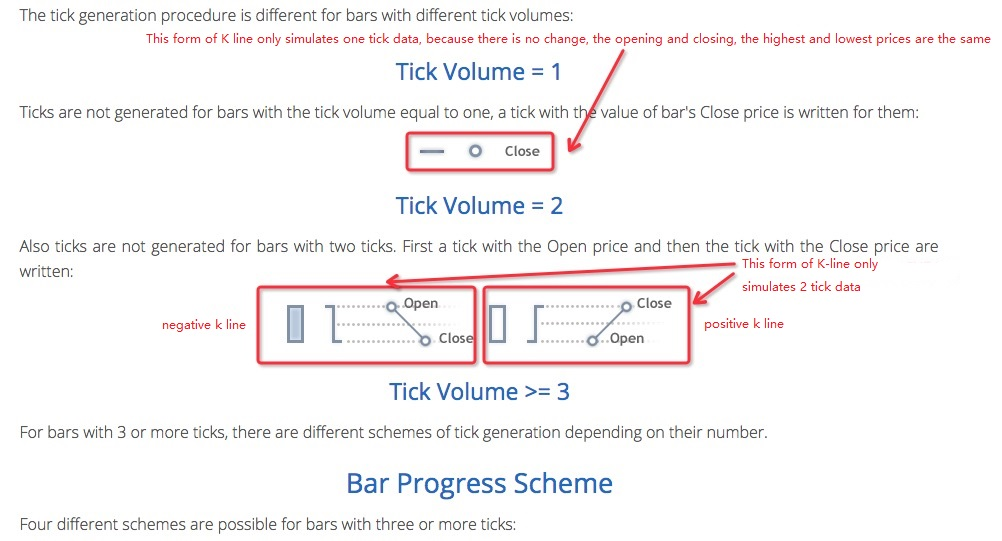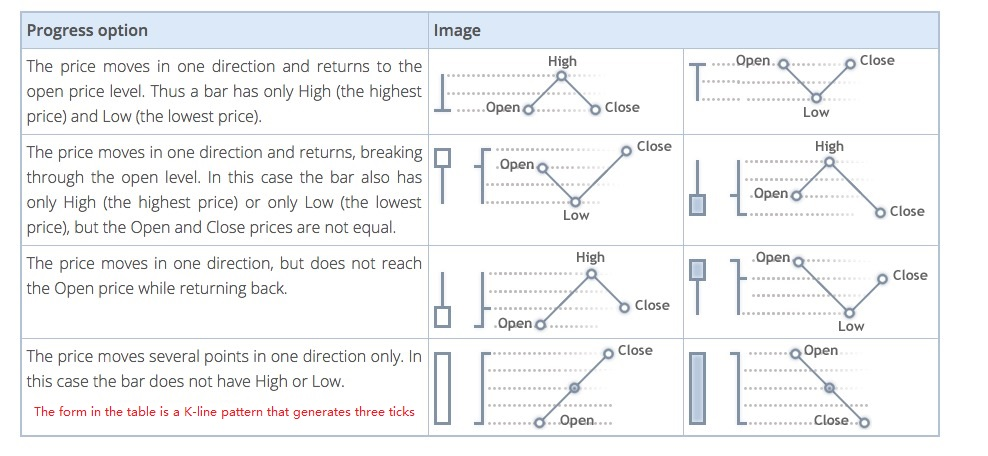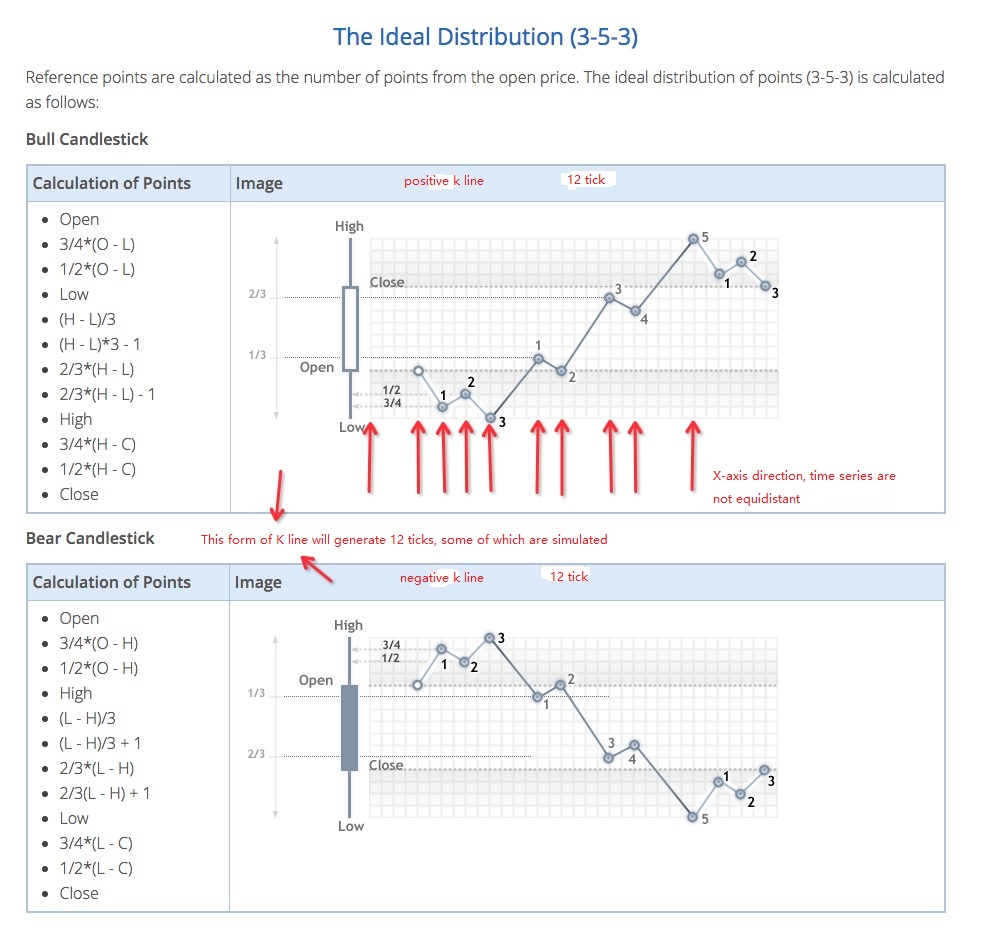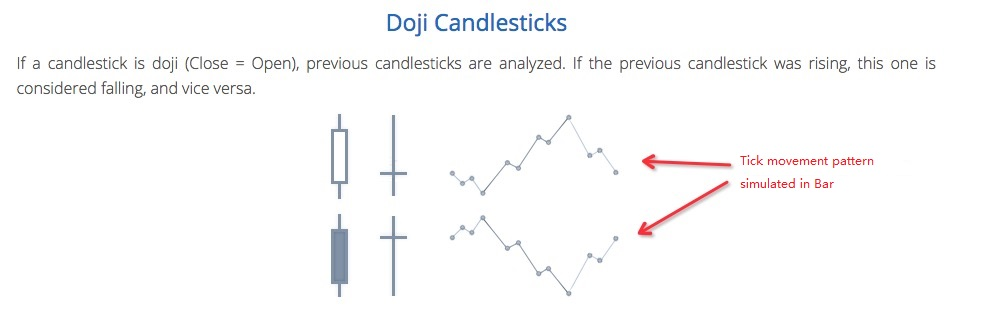# FMZ simulation level backtest mechanism explanation

Author: , Created: 2020-06-04 09:46:50, Updated:

## Backtest architecture

The FMZ platform backtest program is a complete control process, and the program is polling non-stop according to a certain frequency. The data returned by each market and trading API also simulates the actual running time according to the calling time. It belongs to the `onTick` level, not the `onBar` level of other backtest systems. Better support for backtest of strategies based on `Ticker` data (strategies with higher operating frequency).

## The difference between simulation level backtest and real market level backtest

• Simulation level backtest

The simulation level backtest is based on the bottom K-line data of the backtest system, and according to a certain algorithm, the ticker data interpolation is simulated within the framework of the values ​​of the highest, lowest, opening, and closing prices of the given bottom K-line Bar into this `Bar` time series.

• Real market level backtest

The real market level backtest is the real ticker level data in `Bar`'s time series. For strategies based on ticker-level data, using real market level backtest is closer to reality. Backtest at the real market level, ticker is real recorded data, not simulated.

## Simulation level backtest mechanism-bottom K line

There is no bottom K-line option for real market backtest (because ticker data is real, no bottom K-line is needed to simulate the generation).

In the simulation level backtest, the generated `ticker` is simulated based on the K-line data. This K-line data is the bottom K-line. In the actual use of simulation level backtest, the period of the bottom K line must be less than the period of calling the API to obtain the K line when the strategy is running. Otherwise, due to the large cycle of the bottom K line and insufficient number of generated tickers, the data will be distorted when calling the API to obtain the K line of the specified period. When using the large-period K-line backtest, you can appropriately increase the bottom K-line cycle.

## How to generate ticker data for the bottom K line

The mechanism for generating simulated tickers on the bottom K line is the same as the famous trading software MetaTrader 4## Algorithm code for generating ticker data

Specific algorithm for simulating tick data from the bottom K-line data:

``````function recordsToTicks(period, num_digits, records) {
if (records.length == 0) {
return []
}
var ticks = []
var steps = [0, 2, 4, 6, 10, 12, 16, 18, 23, 25, 27, 29]
var pown = Math.pow(10, num_digits)

function pushTick(t, price, vol) {
ticks.push([Math.floor(t), Math.floor(price * pown) / pown, vol])
}

for (var i = 0; i < records.length; i++) {
var T = records[i]
var O = records[i]
var H = records[i]
var L = records[i]
var C = records[i]
var V = records[i]
if (V > 1) {
V = V - 1
}
if ((O == H) && (L == C) && (H == L)) {
pushTick(T, O, V)
} else if (((O == H) && (L == C)) || ((O == L) && (H == C))) {
pushTick(T, O, V)
} else if ((O == C) && ((O == L) || (O == H))) {
pushTick(T, O, V / 2)
pushTick(T + (period / 2), (O == L ? H : L), V / 2)
} else if ((C == H) || (C == L)) {
pushTick(T, O, V / 2)
pushTick(T + (period * 0.382), (C == L ? H : L), V / 2)
} else if ((O == H) || (O == L)) {
pushTick(T, O, V / 2)
pushTick(T + (period * 0.618), (O == L ? H : L), V / 2)
} else {
var dots = []
var amount = V / 11
pushTick(T, O, amount)
if (C > O) {
dots = [
O - (O - L) * 0.75,
O - (O - L) * 0.5,
L,
L + (H - L) / 3.0,
L + (H - L) * (4 / 15.0),
H - (H - L) / 3.0,
H - (H - L) * (6 / 15.0),
H,
H - (H - C) * 0.75,
H - (H - C) * 0.5,
]
} else {
dots = [
O + (H - O) * 0.75,
O + (H - O) * 0.5,
H,
H - (H - L) / 3.0,
H - (H - L) * (4 / 15.0),
H - (H - L) * (2 / 3.0),
H - (H - L) * (9 / 15.0),
L,
L + (C - L) * 0.75,
L + (C - L) * 0.5,
]
}
for (var j = 0; j < dots.length; j++) {
pushTick(T + period * (steps[j + 1] / 30.0), dots[j], amount)
}
}
pushTick(T + (period * 0.98), C, 1)
}
return ticks
}
``````

Therefore, when using the simulation level backtest, there will be price jumps in the time series.

More